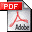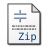Nonnegative signal reconstruction from compressive samples
via a difference map ECME algorithm

K. Qiu and A. Dogandžić

in Proc. IEEE Workshop on Statistical Signal Processing, Nice, France, Jun. 2011, pp. 561-564.

Abstract:
We develop an approximate maximum likelihood (ML) scheme for reconstructing nonnegative sparse signals from compressive samples. The measurements follow an underdetermined linear model, where the regression vector is modeled as the sum of an unknown deterministic nonnegative signal with sparse transform coefficients and a zero-mean white Gaussian component with an unknown variance. We first derive an expectation-conditional maximization either (ECME) algorithm that aims at maximizing the likelihood function with respect to the unknown parameters and then employ a difference map iteration to approximate the maximization (M) step of the ECME iteration. We compare the proposed and existing large-scale sparse signal reconstruction methods via numerical simulations and demonstrate that, by exploiting both the nonnegativity of the underlying image and the sparsity of its wavelet coefficients, we can reconstruct this image using a significantly smaller number of measurements than the existing methods.(377 KB)(Version 1.0, last updated Jun. 17, 2011)